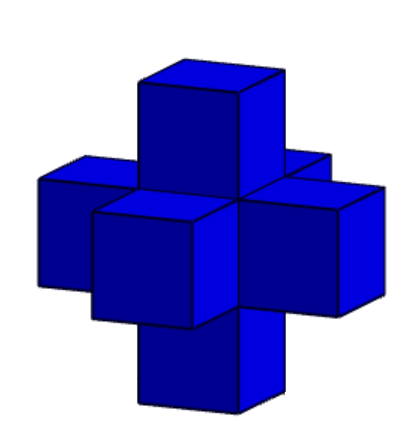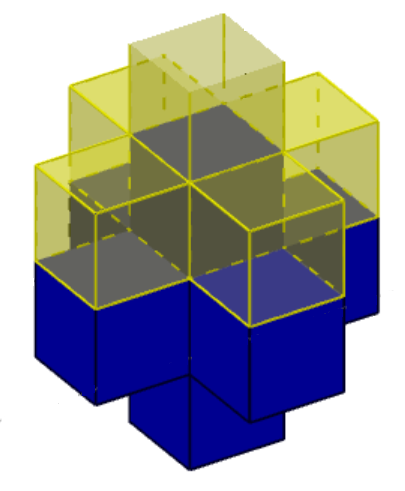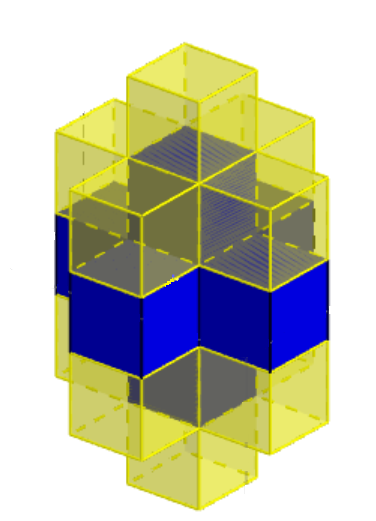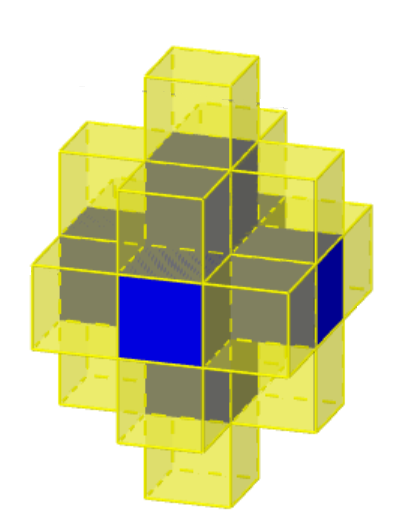#### You may also like### Three Cubes

Can you work out the dimensions of the three cubes?### Nine Colours

Can you use small coloured cubes to make a 3 by 3 by 3 cube so that each face of the bigger cube contains one of each colour?### Marbles in a Box

How many winning lines can you make in a three-dimensional version of noughts and crosses?

# Cubic Covering

##### Age 14 to 16 ShortChallenge Level
We first imagine the cross with the blue cubes only:We now glue the yellow faces to the up and bottom faces of the blue cross. We require 5 yellow cubes for wrapping each of the two blue cubes. (We used transparent yellow cubes)Now we need one yellow cube for each of the four corners:Finally, we have 4 faces to cover, so we need 4 more squares.

Therefore, we used $5+5+4+4 = 18$ yellow cubes.

This problem is taken from the UKMT Mathematical Challenges.
You can find more short problems, arranged by curriculum topic, in our short problems collection.